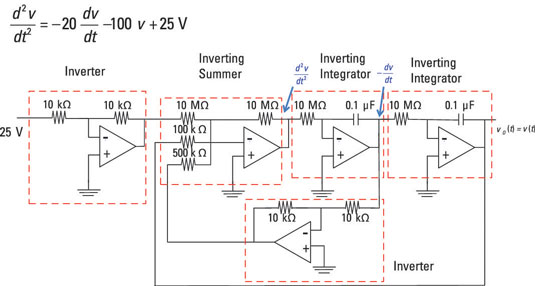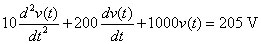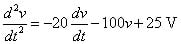##### Circuit Analysis For DummiesThe op amp circuit can solve mathematical equations fast, including calculus problems such as differential equations. To solve a differential equation by finding v(t), for example, you could use various op amp configurations to find the output voltage vo(t) = v(t).

To simplify the problem, assume zero initial conditions: zero initial capacitor voltage for each integrator as shown here. To solve a differential equation, you need to develop a block diagram for the differential equation (which is represented by the dashed boxes in the figure), giving the input and the output for each dashed box. Then use the block diagram to design a circuit.On the far left of the figure is a forcing function of 25 volts derived from the following steps; the output voltage vo(t) = v(t) is on the far right.

Here are the basic steps for designing the circuit:

1. Solve for the highest-order derivative, showing that it consists of a sum of the lower derivatives.

Suppose you want to solve the following second-order differential equation:The first step is to algebraically solve for the highest-order derivative, d2v/dt2:The highest-order derivative is a combination or sum of lower derivatives and the smaller input voltage: dv/dt, v, and 25. Therefore, you need an inverting summer to add the three terms, and these terms are forcing functions (or inputs) to the inverting summer.

2. Use integrators to help implement the block diagram, because the integral of the higher-order derivative is the derivative that’s one order lower.

For this example, integrate the second derivative, d2v/dt2, to give you the first derivative, dv/dt. As shown here, the output of the inverting summing amplifier is the second derivative (which is also the input to the first integrator).

The output of the first inverting integrator is the negative of the first derivative dv/dt and serves as the input to the second inverting integrator. With the second inverting integrator shown in the figure, integrate the negative of the first derivative, –dv/dt, to give you the desired output, v(t).

3. Take the outputs of the integrators, scale them, and feed them back to a summer (summing amplifier).

The second derivative consists of a sum of three terms, so this is where the op amp inverting summer comes in.

1. One of the inputs is a constant of 25 volts to the summer and will be an input voltage (or driving) source. The 25 volts at the input is fed to one of the inputs to the summer with a gain of 1.

2. The output of the first integrator is the first derivative of v(t), which has a weight of 20 and is fed to the second input of the inverting summer.

3. The output of the second integrator is fed to the third input to the inverting summer with a weight of 100.

This completes the block diagram.

For this example, multiply the first derivative dv/dt by –10 and multiply v by –100. Sum them as shown in the block diagram.

4. Design the circuit to implement the block diagram.

To simplify the design, give each integrator a gain of –1. You need two more inverting amplifiers to make the signs come out right. Use the summer to achieve the gains of –10 and –100 found in Step 3.

The sample circuit shown here is one of many possible designs. But you can apply this basic process to solve their differential equations using op amps.